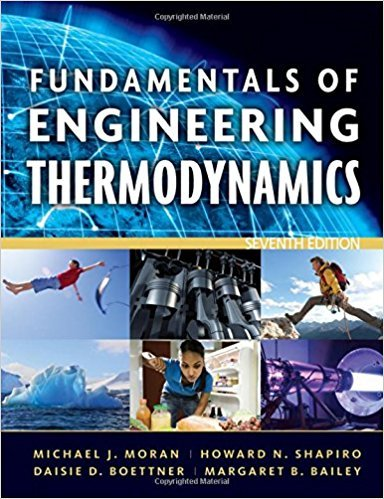×
×

# Solved: Evaluating WorkMeasured data for pressure versusISBN: 9780470495902 50

## Solution for problem 24P Chapter 2

Fundamentals of Engineering Thermodynamics | 7th Edition

• Textbook Solutions
• 2901 Step-by-step solutions solved by professors and subject experts
• Get 24/7 help from StudySoup virtual teaching assistantsFundamentals of Engineering Thermodynamics | 7th Edition

4 5 1 307 Reviews
26
2
Problem 24P

Evaluating Work

Measured data for pressure versus volume during the expansion of gases within the cylinder of an internal combustion engine are given in the table below. Using data from the table, complete the following:

(a) Determine a value of n such that the data are fit by an equation of the form, pVn = constant.

(b) Evaluate analytically the work done by the gases, in kJ, using Eq. 2.17along with the result of part (a).(c) Using graphical or numerical integration of the data, evaluate the work done by the gases, in kJ.

(d) Compare the different methods for estimating the work used in parts (b) and (c). Why are they estimates?

 Data Point p (bar) V (cm3) 1 15 300 2 12 361 3 9 459 4 6 644 5 4 903 6 2 1608

Eq. 2.17

Step-by-Step Solution:

Step 1 of 4</p>

Part a

From the data given, we are required to calculate the value offrom the equationconstant.

Given:constant

Taking logarithm on both sides,,is the constant here…..(1)

Comparing this equation with a straight line equation,will be the slope. If we plot the given data, we can calculate the slope and then the value of.

 Data Point p (bar) V (cm3) 1 15 300 2 12 361 3 9 459 4 6 644 5 4 903 6 2 1608

Convert the above values ofandto logarithmic values and we get the following table.

log (V)                     log (p)

2.47712        1.17609

2.55751        1.07918

2.66181        0.95424

2.80889        0.77815

2.95569        0.60206

3.20629        0.30103

Note thatis taken as the x-axis and log (p) as the y-axis and we get the following plot.Thevsplot is linear fitted in Origin Pro software. The fitting gives us the slope of  -1.19 which is simply the value of.

Therefore, the value ofis 1.19.

Step 2 of 4</p>

Part b

Equation 2.17 can be written as,…..(1)

From the table,barN/m2barN/m2cm3m3m3

Substitute these values in equation (1),JJkJ

This is the required work done.

Step 3 of 3

##### ISBN: 9780470495902

Since the solution to 24P from 2 chapter was answered, more than 1445 students have viewed the full step-by-step answer. This textbook survival guide was created for the textbook: Fundamentals of Engineering Thermodynamics, edition: 7. The full step-by-step solution to problem: 24P from chapter: 2 was answered by , our top Engineering and Tech solution expert on 07/20/17, 09:01AM. This full solution covers the following key subjects: data, work, gases, Using, evaluate. This expansive textbook survival guide covers 14 chapters, and 1501 solutions. The answer to “Evaluating WorkMeasured data for pressure versus volume during the expansion of gases within the cylinder of an internal combustion engine are given in the table below. Using data from the table, complete the following:(a) Determine a value of n such that the data are fit by an equation of the form, pVn = constant.________________(b) Evaluate analytically the work done by the gases, in kJ, using Eq. 2.17along with the result of part (a). ________________(c) Using graphical or numerical integration of the data, evaluate the work done by the gases, in kJ.________________(d) Compare the different methods for estimating the work used in parts (b) and (c). Why are they estimates?Data Pointp (bar)V (cm3)115300212361394594664454903621608Eq. 2.17” is broken down into a number of easy to follow steps, and 113 words. Fundamentals of Engineering Thermodynamics was written by and is associated to the ISBN: 9780470495902.

Unlock Textbook Solution